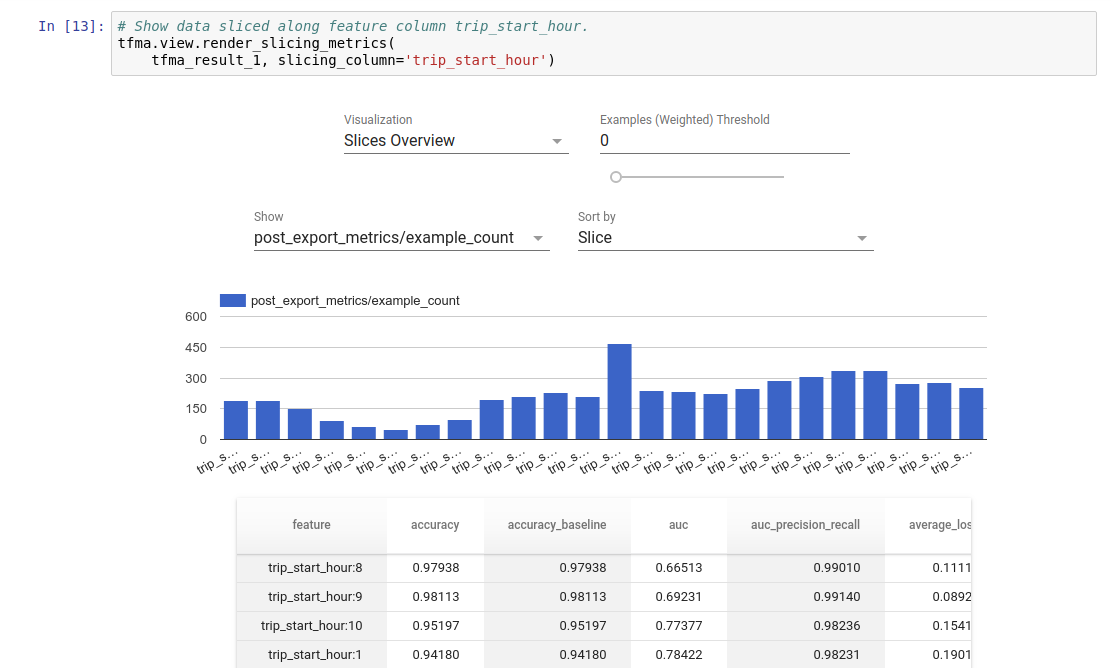# Get Started with TensorFlow Model Analysis

TensorFlow Model Analysis (TFMA) can export a model's evaluation graph to a special `SavedModel` called `EvalSavedModel`. (Note that the evaluation graph is used and not the graph for training or inference.) The `EvalSavedModel` contains additional information that allows TFMA to compute the same evaluation metrics defined in the model in a distributed manner over a large amount of data and user-defined slices.

## Modify an existing model

To use an existing model with TFMA, first modify the model to export the `EvalSavedModel`. This is done by adding a call to `tfma.export.export_eval_savedmodel` and is similar to `estimator.export_savedmodel`. For example:

``````# Define, train and export your estimator as usual
estimator = tf.estimator.DNNClassifier(...)
estimator.train(...)
estimator.export_savedmodel(...)

# Also export the EvalSavedModel
tfma.export.export_eval_savedmodel(
estimator=estimator, export_dir_base=export_dir,
``````

`eval_input_receiver_fn` must be defined and is similar to the `serving_input_receiver_fn` for `estimator.export_savedmodel`. Like `serving_input_receiver_fn`, the `eval_input_receiver_fn` function defines an input placeholder example, parses the features from the example, and returns the parsed features. It parses and returns the label.

The following snippet defines an example `eval_input_receiver_fn`:

``````country = tf.feature_column.categorical_column_with_hash('country', 100)
language = tf.feature_column.categorical_column_with_hash('language', 100)
age = tf.feature_column.numeric_column('age')
label = tf.feature_column.numeric_column('label')

serialized_tf_example = tf.compat.v1.placeholder(
dtype=tf.string, shape=[None], name='input_example_placeholder')

# This *must* be a dictionary containing a single key 'examples', which
# points to the input placeholder.

feature_spec =  tf.feature_column.make_parse_example_spec(
[country, language, age, label])
features = tf.io.parse_example(serialized_tf_example, feature_spec)

features=features,
labels=features['label'])
``````

In this example you can see that:

• `labels` can also be a dictionary. Useful for a multi-headed model.
• The `eval_input_receiver_fn` function will, most likely, be the same as your `serving_input_receiver_fn` function. But, in some cases, you may want to define additional features for slicing. For example, you introduce an `age_category` feature which divides the `age` feature into multiple buckets. You can then slice on this feature in TFMA to help understand how your model's performance differs across different age categories.

## Use TFMA to evaluate the modified model

TFMA can perform large-scale distributed evaluation of your model by using Apache Beam, a distributed processing framework. The evaluation results can be visualized in a Jupyter notebook using the frontend components included in TFMA.Use `tfma.run_model_analysis` for evaluation. Since this uses Beam's local runner, it's mainly for local, small-scale experimentation. For example:

``````# Note that this code should be run in a Jupyter Notebook.

# This assumes your data is a TFRecords file containing records in the format
# your model is expecting, e.g. tf.train.Example if you're using
eval_shared_model = tfma.default_eval_shared_model(
eval_saved_model_path='/path/to/eval/saved/model')
eval_result = tfma.run_model_analysis(
eval_shared_model=eval_shared_model,
data_location='/path/to/file/containing/tfrecords',
file_format='tfrecords')

tfma.view.render_slicing_metrics(eval_result)
``````

Compute metrics on slices of data by configuring the `slice_spec` parameter. Add additional metrics that are not included in the model with `add_metrics_callbacks`. For more details, see the Python help for `run_model_analysis`.

For distributed evaluation, construct an Apache Beam pipeline using a distributed runner. In the pipeline, use the `tfma.ExtractEvaluateAndWriteResults` for evaluation and to write out the results. The results can be loaded for visualization using `tfma.load_eval_result`. For example:

``````# To run the pipeline.
eval_shared_model = tfma.default_eval_shared_model(
model_path='/path/to/eval/saved/model')
with beam.Pipeline(runner=...) as p:
_ = (p
# You can change the source as appropriate, e.g. read from BigQuery.
| 'ExtractEvaluateAndWriteResults' >>
tfma.ExtractEvaluateAndWriteResults(
eval_shared_model=eval_shared_model,
output_path='/path/to/output',
display_only_data_location=data_location))

# To load and visualize results.
# Note that this code should be run in a Jupyter Notebook.